# Maharashtra Board 12th Maths Solutions Chapter 6 Line and Plane Ex 6.3

Balbharti 12th Maharashtra State Board Maths Solutions Book Pdf Chapter 6 Line and Plane Ex 6.3 Questions and Answers.

## Maharashtra State Board 12th Maths Solutions Chapter 6 Line and Plane Ex 6.3

Question 1.
Find the vector equation of a plane which is at 42 unit distance from the origin and which is normal to the vector $$2 \hat{i}+\hat{j}-2 \hat{k}$$.
Solution:
If $$\hat{n}$$ is a unit vector along the normal and p is the length of the perpendicular from origin to the plane, then the vector equation of the plane is $$\bar{r} \cdot \hat{n}$$ = p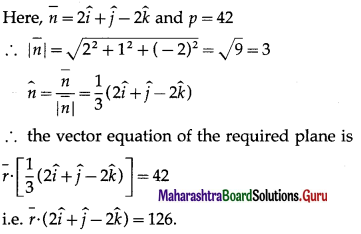Question 2.
Find the perpendicular distance of the origin from the plane 6x – 2y + 3z – 7 = 0.
Solution:
The equation of the plane is
6x – 2y + 3z – 7 = 0
∴ its vector equation is
$$\bar{r} \cdot(6 \hat{i}-2 \hat{j}+3 \hat{k})$$ = 7 ….(1)
where $$\bar{r}=x \hat{i}+y \hat{j}+z \hat{k}$$
∴ $$\bar{n}=6 \hat{i}-2 \hat{j}+3 \hat{k}$$ is normal to the plane
$$|\bar{n}|=\sqrt{6^{2}+(-2)^{2}+3^{2}}=\sqrt{49}$$ = 7
Unit vector along $$\bar{n}$$ is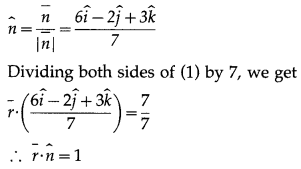Comparing with normal form of equation of the plane $$\bar{r} \cdot \hat{n}$$ = p, it follows that length of perpendicular from origin is 1 unit.
Alternative Method:
The equation of the plane is 6x – 2y + 3z – 7 = 0 i.e. 6x – 2y + 3z = 7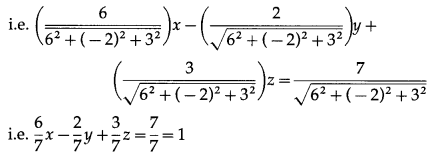This is the normal form of the equation of plane.
∴ perpendicular distance of the origin from the plane is p = 1 unit.Question 3.
Find the coordinates of the foot of the perpendicular drawn from the origin to the plane 2x + 6y – 3z = 63 .
Solution:
The equation of the plane is 2x + 6y – 3z = 63. Dividing each term by $$\sqrt{2^{2}+6^{2}+(-3)^{2}}=\sqrt{49}$$ = 7, we get
$$\frac{2}{7} x+\frac{6}{7} y-\frac{3}{7} z=\frac{63}{7}$$ = 9
This is the normal form of the equation of plane.
∴ the direction cosines of the perpendicular drawn from the origin to the plane are
l = $$\frac{2}{7}$$, m = $$\frac{6}{7}$$, n = $$-\frac{3}{7}$$
and length of perpendicular from origin to the plane is p = 9.
∴ the coordinates of the foot of the perpendicular from the origin to the plane are (lp, mp, np) i.e. $$\left(\frac{18}{7}, \frac{54}{7},-\frac{27}{7}\right)$$.

Question 4.
Reduce the equation $$\bar{r} \cdot(3 \hat{i}+4 \hat{j}+12 \hat{k})$$ = 78 to normal form and hence find
(i) the length of the perpendicular from the origin to the plane
(ii) direction cosines of the normal.
Solution:
The normal form of equation of a plane is $$\bar{r} \cdot \hat{n}$$ = p where $$\hat{n}$$ is unit vector along the normal and p is the length of perpendicular drawn from origin to the plane.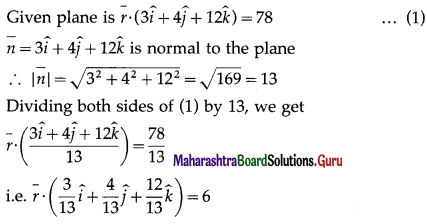This is the normal form of the equation of plane. Comparing with $$\bar{r} \cdot \hat{n}$$ = p,
(i) the length of the perpendicular from the origin to plane is 6.
(ii) direction cosines of the normal are $$\frac{3}{13}, \frac{4}{13}, \frac{12}{13}$$.Question 5.
Find the vector equation of the plane passing through the point having position vector $$\hat{i}+\hat{j}+\hat{k}$$ and perpendicular to the vector $$4 \hat{i}+5 \hat{j}+6 \hat{k}$$.
Solution:
The vector equation of the plane passing through the point A ($$\bar{a}$$) and perpendicular to the vector $$\bar{n}$$ is $$\bar{r} \cdot \bar{n}=\bar{a} \cdot \bar{n}$$
Here, $$\bar{a}=\hat{i}+\hat{j}+\hat{k}$$, $$\bar{n}=4 \hat{i}+5 \hat{j}+6 \hat{k}$$
∴ $$\bar{a} \cdot \bar{n}$$ = $$(\hat{i}+\hat{j}+\hat{k}) \cdot(4 \hat{i}+5 \hat{j}+6 \hat{k})$$
= (1)(4) + (1)(5) + (1)(6)
= 4 + 5 + 6 = 15
∴ the vector equation of the required plane is $$\bar{r} \cdot(4 \hat{i}+5 \hat{j}+6 \hat{k})$$ = 15.

Question 6.
Find the Cartesian equation of the plane passing through A( -1, 2, 3), the direction ratios of whose normal are 0, 2, 5.
Solution:
The cartesian equation of the plane passing ; through (x1, y1, z1), the direction ratios of whose normal are a, b, c, is
a(x – x1) + b(y – y1) + c(z – z1) = 0
∴ the cartesian equation of the required plane is
0(x +1) + 2(y – 2) + 5(z – 3) = 0
i.e. 0 + 2y – 4 + 5z – 15 = 0
i.e. 2y + 5z = 19.Question 7.
Find the Cartesian equation of the plane passing through A(7, 8, 6) and parallel to the XY plane.
Solution:
The cartesian equation of the plane passing through (x1, y1, z1), the direction ratios of whose normal are a, b, c, is
a(x – x1) + b(y – y1) + c(z – z1) = 0
The required plane is parallel to XY-plane.
∴ it is perpendicular to Z-axis i.e. Z-axis is normal to the plane. Z-axis has direction ratios 0, 0, 1.
The plane passes through (7, 8, 6).
∴ the cartesian equation of the required plane is
0(x – 7) + 0(y – 8) + 1 (z – 6) = 0
i.e. z = 6.

Question 8.
The foot of the perpendicular drawn from the origin to a plane is M(1, 0, 0). Find the vector equation of the plane.
Solution:
The vector equation of the plane passing ; through A($$\bar{a}$$) and perpendicular to $$\bar{n}$$ is $$\bar{r} \cdot \bar{n}=\bar{a} \cdot \bar{n}$$.
M(1, 0, 0) is the foot of the perpendicular drawn from ; origin to the plane. Then the plane is passing through M : and is perpendicular to OM.
If $$\bar{m}$$ is the position vector of M, then $$\bar{m}$$ = $$\hat{i}$$
Normal to the plane is
$$\bar{n}$$ = $$\overline{\mathrm{OM}}$$ = $$\hat{i}$$
$$\bar{m} \cdot \bar{n}=\hat{i} \cdot \hat{i}$$ = 1
∴ the vector equation of the required plane is $$\bar{r} \cdot \hat{i}$$ = 1Question 9.
Find the vector equation of the plane passing through the point A(-2, 7, 5) and parallel to vectors $$\hat{4}-\hat{j}+3 \hat{k}$$ and $$\hat{i}+\hat{j}+\hat{k}$$.
Solution:
The vector equation of the plane passing through the point A($$\bar{a}$$) and parallel to the vectors $$\bar{b}$$ and $$\bar{c}$$ is
$$\bar{r} \cdot(\bar{b} \times \bar{c})=\bar{a} \cdot(\bar{b} \times \bar{c})$$ ….(1)Question 10.
Find the Cartesian equation of the plane $$\bar{r}=(5 \hat{i}-2 \hat{j}-3 \hat{k})+\lambda(\hat{i}+\hat{j}+\hat{k})+\mu(\hat{i}-2 \hat{j}+3 \hat{k})$$
Solution:
The equation  represents a plane passing through a point having position vector $$\bar{a}$$ and parallel to vectors $$\bar{b}$$ and $$\bar{c}$$.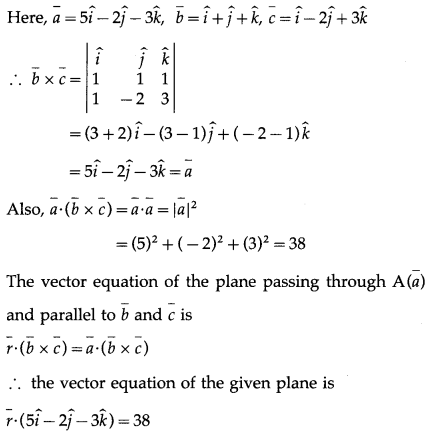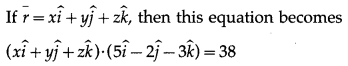∴ 5x – 2y – 3z = 38.
This is the cartesian equation of the required plane.Question 11.
Find the vector equation of the plane which makes intercepts 1, 1, 1 on the co-ordinates axes.
Solution:
The vector equation of the plane passing through A($$\bar{a}$$), B($$\bar{b}$$). C($$\bar{c}$$), where A, B, C are non-collinear is $$\bar{r} \cdot(\overline{\mathrm{AB}} \times \overline{\mathrm{AC}})$$ = $$\bar{a} \cdot(\overline{\mathrm{AB}} \times \overline{\mathrm{AC}})$$ … (1)
The required plane makes intercepts 1, 1, 1 on the coordinate axes.
∴ it passes through the three non-collinear points A (1, 0, 0), B = (0, 1, 0), C = (0, 0, 1)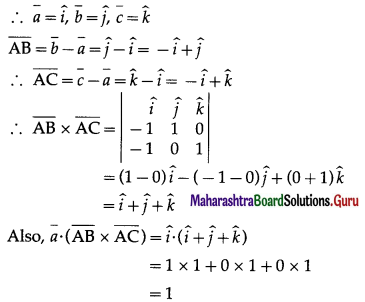∴ from (1), the vector equation of the required plane is $$\bar{r} \cdot(\hat{i}+\hat{j}+\hat{k})$$ = 1.